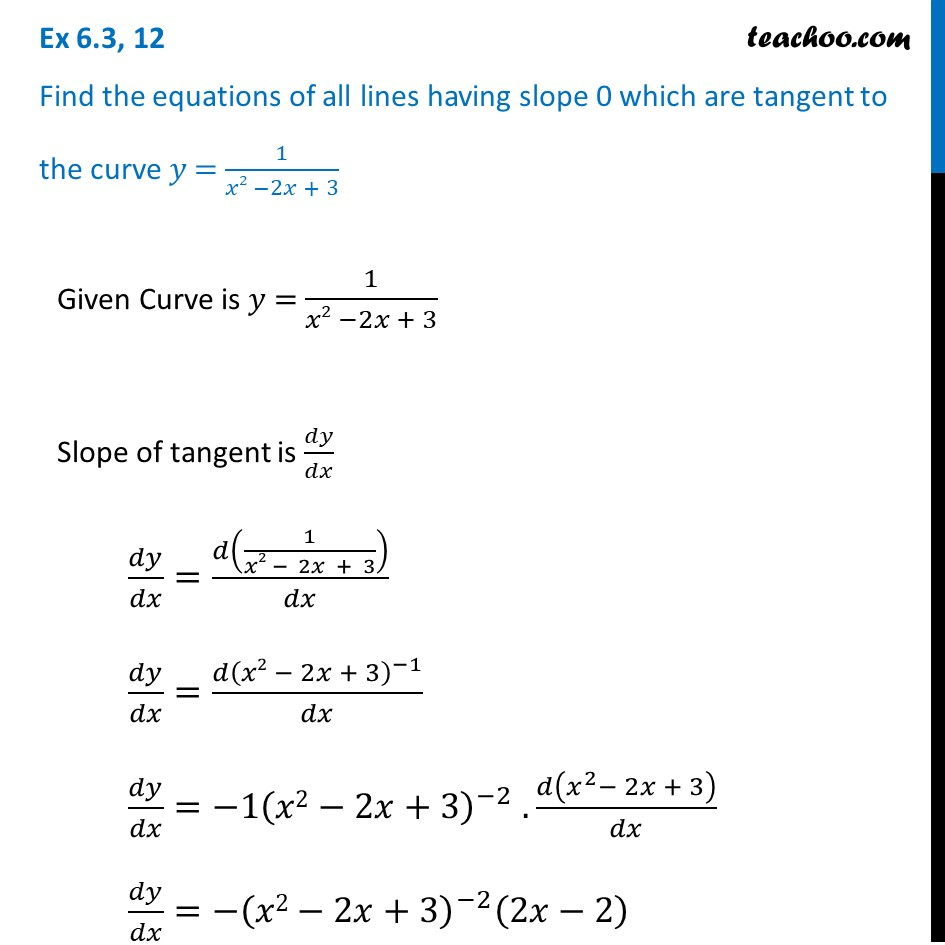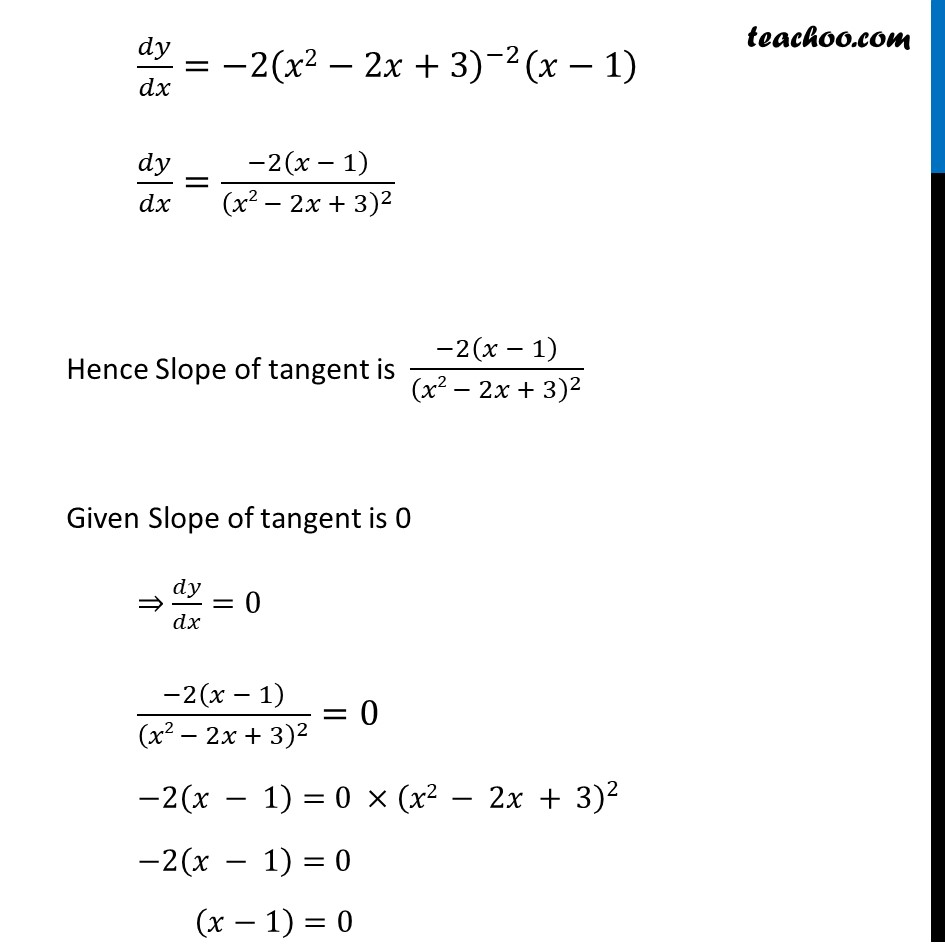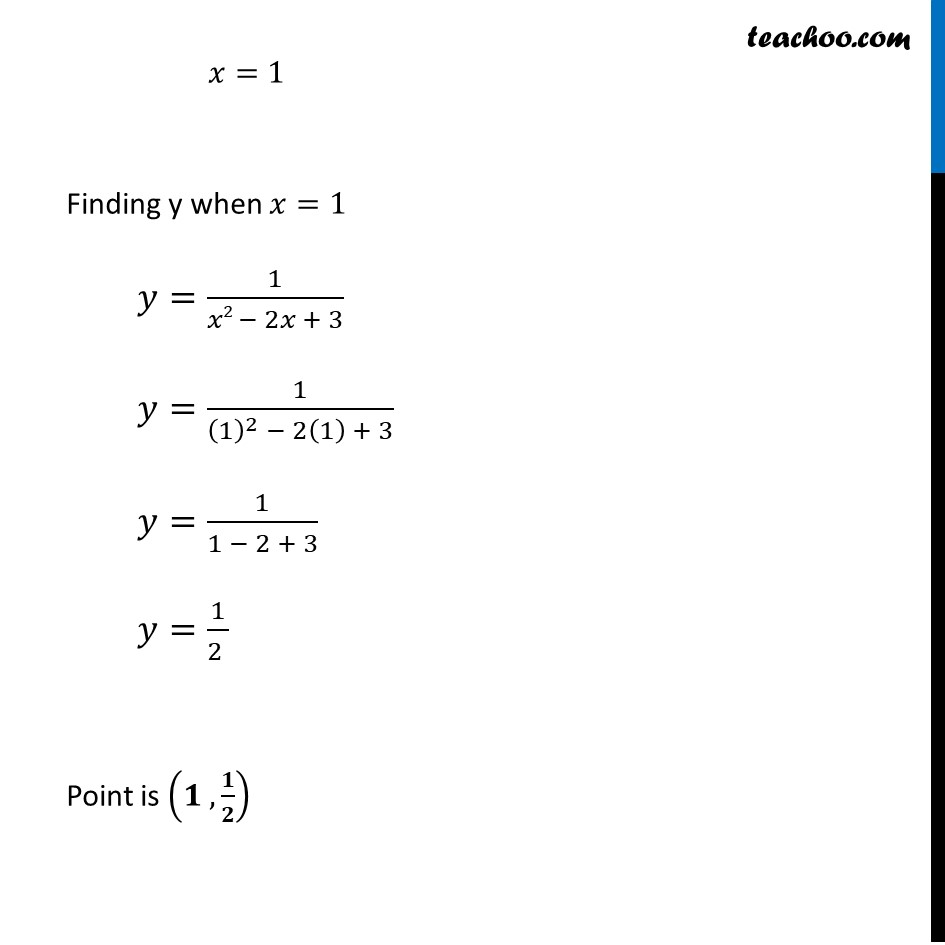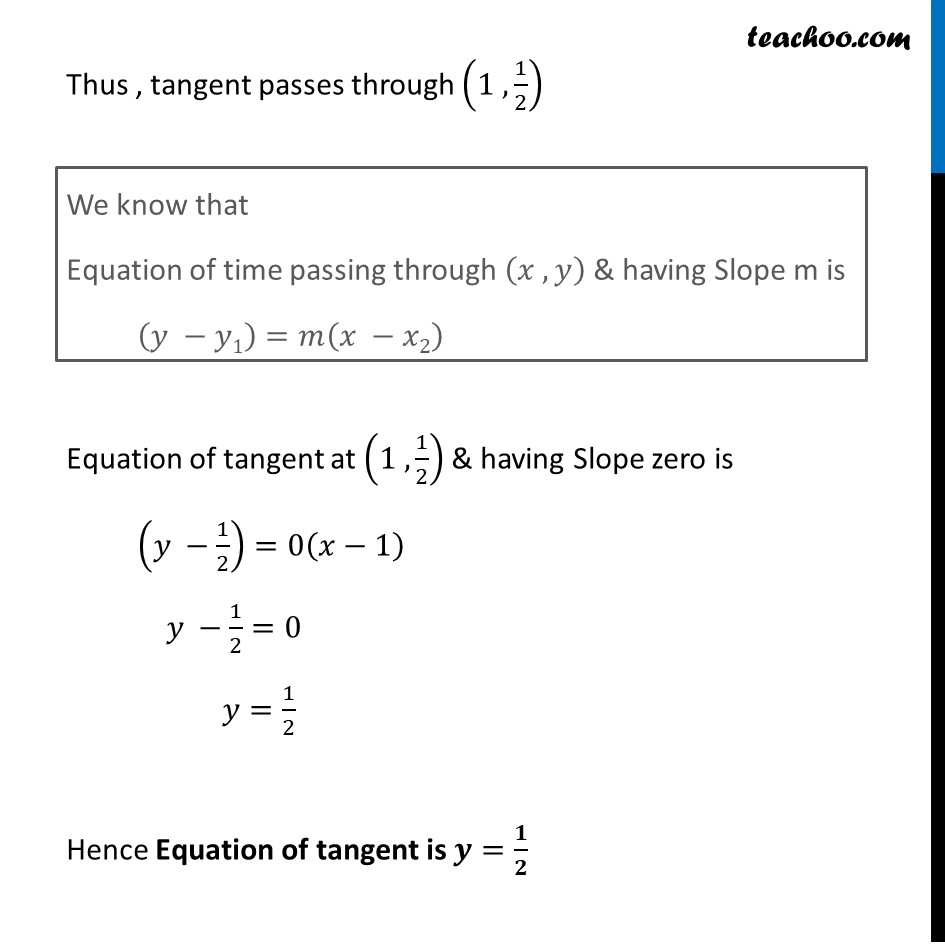Tangents and Normals (using Differentiation)

Chapter 6 Class 12 Application of Derivatives
Serial order wiseLearn in your speed, with individual attention - Teachoo Maths 1-on-1 Class

### Transcript

Question 12 Find the equations of all lines having slope 0 which are tangent to the curve 𝑦 = 1/(𝑥2 −2𝑥 + 3) Given Curve is 𝑦 = 1/(𝑥2 −2𝑥 + 3) Slope of tangent is 𝑑𝑦/𝑑𝑥 𝑑𝑦/𝑑𝑥=𝑑(1/(𝑥2 − 2𝑥 + 3))/𝑑𝑥 𝑑𝑦/𝑑𝑥=(𝑑(𝑥2 − 2𝑥 + 3)^(−1))/𝑑𝑥 𝑑𝑦/𝑑𝑥=−1(𝑥2−2𝑥+3)^(−2) . 𝑑(𝑥^2− 2𝑥 + 3)/𝑑𝑥 𝑑𝑦/𝑑𝑥=−(𝑥2−2𝑥+3)^(−2) (2𝑥−2) 𝑑𝑦/𝑑𝑥=−2(𝑥2−2𝑥+3)^(−2) (𝑥−1) 𝑑𝑦/𝑑𝑥=(−2(𝑥 − 1))/(𝑥2 − 2𝑥 + 3)^2 Hence Slope of tangent is (−2(𝑥 − 1))/(𝑥2 − 2𝑥 + 3)^2 Given Slope of tangent is 0 ⇒ 𝑑𝑦/𝑑𝑥=0 (−2(𝑥 − 1))/(𝑥2 − 2𝑥 + 3)^2 =0 −2(𝑥 − 1)=0 ×(𝑥2 − 2𝑥 + 3)^2 −2(𝑥 − 1)=0 (𝑥−1)=0 𝑥=1 Finding y when 𝑥=1 𝑦=1/(𝑥2 − 2𝑥 + 3) 𝑦=1/((1)^2 − 2(1) + 3) 𝑦=1/(1 − 2 + 3) 𝑦=1/(2 ) Point is (𝟏 , 𝟏/𝟐) Thus , tangent passes through (1 , 1/2) Equation of tangent at (1 , 1/2) & having Slope zero is (𝑦 −1/2)=0(𝑥−1) 𝑦 −1/2=0 𝑦=1/2 Hence Equation of tangent is 𝒚=𝟏/𝟐 We know that Equation of time passing through (𝑥 , 𝑦) & having Slope m is (𝑦 −𝑦1)=𝑚(𝑥 −𝑥2)